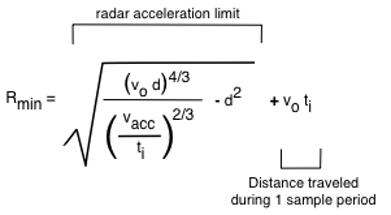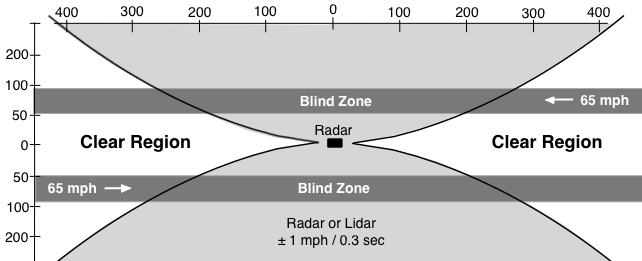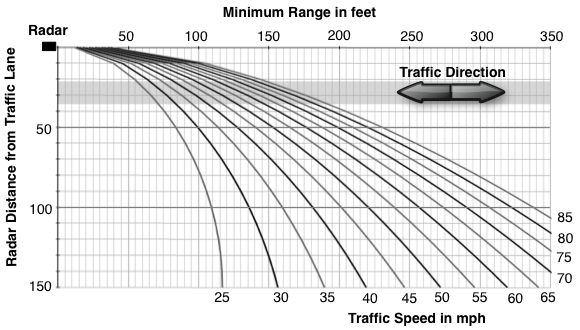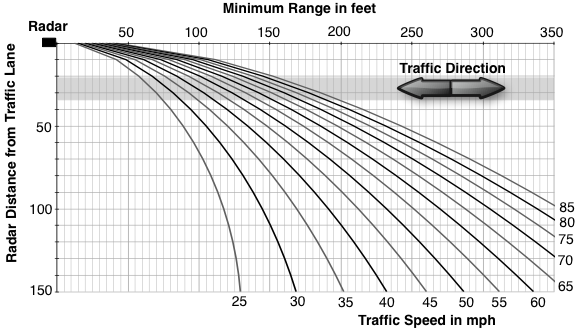Previous | Next

# Cosine Effect Minimum RangeRadar and Lidar

 Cosine Effect Acceleration

The cosine effect induces a deceleration component (-a) for approaching vehicles, and an acceleration component (+a) for receding vehicles. The closer the vehicle the faster the angle and measured speed change and the greater the acceleration component.

The cosine effect acceleration component is a function of vehicle speed and range from radar, and radar distance from vehicle lane. Applying a little calculus and trigonometry to velocity vectors and the geometry the vehicle cosine effect acceleration can be calculated.

Acceleration based on target vehicle speed and range from radar,
and radar distance from target vehicle lane.a = accelerationvo = speed d = radar distance from target vehicle lane centerR = range

The cosine effect equation for acceleration can be used to determine the range for a given acceleration and radar distance from vehicle lane and vehicle speed.

Range based on target vehicle speed, radar distance
from target vehicle lane, and acceleration.a = cosine effect accelerationvo = speed d = radar distance from target vehicle lane centerR = range from radar

The above equations can be used to determine radar minimum range, vehicles closer than minimum range cannot be measured.

 Minimum Range

A radar cannot measure speed when acceleration exceeds the radar limits due to the cosine effect, or anything else - curves, vehicle slowing or accelerating. Radar acceleration limit is a function of radar accuracy and sample time - minimum time required to get one speed measurement. Speed cannot change faster than radar or lidar accuracy during one sample period.

 amax = ±vacc / ti
 amax = radar acceleration limit vacc = radar accuracy ti = radar sample time or integration period

The acceleration limit sets a minimum range, vehicles inside minimum range are too close to measure because the relative speed is changing too fast. Minimum range applies to vehicles traveling toward and away from the radar.

 Minimum Range Factors Traffic Speed Antenna Distance from Traffic Lane Radar Accuracy (Typically ±1 mph) Radar Sample TIme (Typically 0.3 seconds)

Accuracy is typically ±1 mph, sample times vary with model from 1/4 to 1/2 second. Many microwave radars have a sample time of 0.3 seconds (300 milliseconds), laser radars typically have longer sample periods.

Target vehicle acceleration must be within limits for an entire sample time. Radar / lidar minimum range is the range acceleration exceeds limits plus one sample period.

Minimum Range EquationRmin = Minimum Rangevo = Speed vacc = Radar Accuracyd = Radar Distance from Target Vehicle Lane Centerti = Radar Sample Time

Minimum Range Example
Distances in FeetMinimum Range
0.250 second Sample Time, ±1 mph AccuracyMinimum Range
0.300 second Sample Time, ±1 mph AccuracyMinimum Range
0.333 second Sample Time, ±1 mph AccuracyMinimum Range
Traffic closer than minimum range cannot be measured.
 vo = Traffic Speed: mph d = Radar to Traffic Lane: feet ti = Radar Sample Time: seconds milliseconds vacc = Radar Accuracy: ± 1 mph Minimum RangeTime to Even with Radar